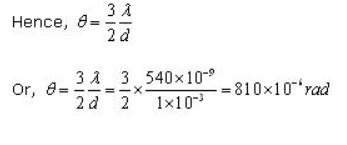Courses

# Test: Diffraction Of Light

## 10 Questions MCQ Test Physics Class 12 | Test: Diffraction Of Light

Description
This mock test of Test: Diffraction Of Light for JEE helps you for every JEE entrance exam. This contains 10 Multiple Choice Questions for JEE Test: Diffraction Of Light (mcq) to study with solutions a complete question bank. The solved questions answers in this Test: Diffraction Of Light quiz give you a good mix of easy questions and tough questions. JEE students definitely take this Test: Diffraction Of Light exercise for a better result in the exam. You can find other Test: Diffraction Of Light extra questions, long questions & short questions for JEE on EduRev as well by searching above.
QUESTION: 1

### Diffraction pattern cannot be observed with:

Solution:

For diffraction, the width of the slit must be less than the wavelength of the incident ray.

QUESTION: 2

### Diffraction of light gives the information of:

Solution:

Diffraction of light takes place for all types of waves transverse or longitudinal.

QUESTION: 3

### In a single slit experiment, suppose the slit width is equal to the wavelength of light used, then:

Solution:

This is because for maximum diffraction the slit width always equals to the wavelength of light.

QUESTION: 4

Light of wavelength 500 nm is incident on a slit of width 0.1 mm. The width of the central bright line on the screen is 2m. What is the distance of the screen?​

Solution:

Beta(central Maxima) = 2 * lambda * D(distance of the screen) / d(distance between slits)
beta = 2 m, wavelength = 500nm = 5 ×10-7m & d = 0.1mm = 1×10-4m
► 2 = 2 × 5 × 10-7 * D/10-4
► 1 = 5 × 10-3
► D = 1000/5
► D = 200m

QUESTION: 5

The phenomenon of diffraction can take place in sound waves?

Solution:
• Yes, sound waves are longitudinal, meaning that they oscillate parallel to the direction of their motion. So diffraction takes place in the sound waves.
• Since there is no component of a sound wave's oscillation that is perpendicular to its motion, sound waves cannot be polarized.
QUESTION: 6

What is the angular spread of the first minimum and the central maximum when diffraction is observed with 540 nm light through a slit of width 1mm?​

Solution:

The First minimum is located at the place where the central maximum ends. And the first minimum spreads further upto half the fringe width.QUESTION: 7

The change in the diffraction pattern of a single slit, when the monochromatic source of light is replaced by a source of white light will be:

Solution:
• When a source of white light is used instead of a monochromatic source, the diffracted image of the slit gets dispersed into constituent colours of white light.
• The central maximum will be white and on either side of the central maximum, there will be coloured fringes.
QUESTION: 8

The diffraction of light is not easily noticed if:

Solution:

Actually, diffraction occurs all the time. But when the aperture/wavelength ratio is large, the spacing between fringes becomes too small, and the diffractive effects are unnoticeable.

QUESTION: 9

In the diffraction of a single slit experiment, if the slit width is half then the width of the central maxima will be:

Solution:

Fringe width is inversely proportional to the distance between slits so it will be doubled.

QUESTION: 10

Which of the following undergoes the largest diffraction?

Solution:
• Diffraction occurs with all waves, including sound waves, water waves, and electromagnetic waves such as visible light, X-rays and radio waves. For the diffraction of wave, an obstacle or aperture of the size of the wavelength of the wave is needed.
• Since the wavelength of the radio waves is large and comparable with the size of the obstacles around which they bend. So it shows maximum diffraction.